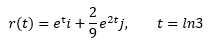# Particle's Equation, Velocity and Acceleration

In summary, you need to find an equation of the form ##y = F(x)## or ##x = G(y)## or ##H(x,y) = 0##, where ##F## or ##G## or ##H## are the functions that represent the curve of the orbit.

## Homework Statement

r(t) is the position of a particle in the xy-plane at time t. Find an equation in x and y whose graph is the path of the particle. Then find the particle’s velocity and acceleration vectors at the given value of t.## Homework Equations

First derivative = velocity
(velocity=distance/time)
Second derivative = acceleration
(acceleration=velocity/time)

## The Attempt at a Solution

To find the Equation, I first organize it into a set:
[ et, 2/9 e2t ]
Then I just plug in the value of t (ln3)
[ eln3, 2/9 e2(ln3) ]
I then reconstruct the original problem with the new values:
r(t) = eln3 i + 2/9 e2(ln3) j
r(ln3) = e1.0986 i + 2/9 e2.1972 j
then I change the i / j to x/y
r(ln3) = e1.0986 x + 2/9 e2.1972 y
-----------------------------

As for velocity and acceleration, so far I have figured it like this:
Velocity:
[ et, 2/9 e2t ]
[ te, 4/9 et ]
Velocity = tei + 4/9etj

Acceleration:
[ e, 4/9 te]
Acceleration = tei + 4/9 tej
Am I taking the derivative correctly? As far as I know, e remains as e, even after the derivative, right?

## Homework Statement

r(t) is the position of a particle in the xy-plane at time t. Find an equation in x and y whose graph is the path of the particle. Then find the particle’s velocity and acceleration vectors at the given value of t.
View attachment 101452

## Homework Equations

First derivative = velocity
(velocity=distance/time)
Second derivative = acceleration
(acceleration=velocity/time)

## The Attempt at a Solution

To find the Equation, I first organize it into a set:
[ et, 2/9 e2t ]
Then I just plug in the value of t (ln3)
[ eln3, 2/9 e2(ln3) ]
I then reconstruct the original problem with the new values:
r(t) = eln3 i + 2/9 e2(ln3) j
r(ln3) = e1.0986 i + 2/9 e2.1972 j
then I change the i / j to x/y
r(ln3) = e1.0986 x + 2/9 e2.1972 y
-----------------------------

As for velocity and acceleration, so far I have figured it like this:
Velocity:
[ et, 2/9 e2t ]
[ te, 4/9 et ]
Velocity = tei + 4/9etj

Acceleration:
[ e, 4/9 te]
Acceleration = tei + 4/9 tej
Am I taking the derivative correctly? As far as I know, e remains as e, even after the derivative, right?

For the derivatives of et, you should review the rules of differentiation for such functions and not neglect application of the chain rule.

http://www.themathpage.com/acalc/exponential.htm

Remember, et is not differentiated like xn.

## Homework Statement

r(t) is the position of a particle in the xy-plane at time t. Find an equation in x and y whose graph is the path of the particle. Then find the particle’s velocity and acceleration vectors at the given value of t.
View attachment 101452

You have not done the second part, which says "Find an equation in x and y whose graph is the path of the particle". This means that instead of representing the particle's orbit as ##(x(t),y(t))## you should represent the curve of the orbit (not including "time" information) as an equation of the form ##y = F(x)## or ##x = G(y)## or ##H(x,y) = 0##, and you are to figure out the functions ##F## or ##G## or ##H##, as needed.

Got it! Thanks for your guys' help!